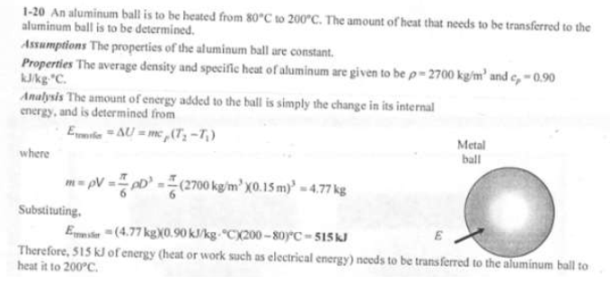heat and mass transfer problems engineering equations heat and mass transfer school homework engineering heat and mass transfer formulas heat and mass transfer solutions to heat and mass transfer problems full solution engineering problem solution heat and mass transfer math problems engineering equations heat and mass transfer school homework engineering solutions to heat and mass transfer formulas heat problems mass problem solutions to transfer problems full solution heat and mass transfer
heat and mass transfer problems engineering equations heat and mass transfer school homework engineering heat and mass transfer formulas heat and mass transfer solutions to heat and mass transfer problems full solution engineering problem solution heat and mass transfer math problems engineering equations heat and mass transfer school homework engineering solutions to heat and mass transfer formulas heat problems mass problem solutions to transfer problems full solution heat and mass transfer
Highalphabet Home Page heat and mass transfer problem solutions Heat and Mass Transfer Page
A 15-cm-diameter aluminum ball is to be heated from 80 C to an average temperature of 200 C. Taking the average density and specific heat of aluminum in this temperature range to be rho=2700 kg/m^3 and c_p=0.90 kJ/kgK, respectively, determine the amount of energy that needs to be transferred to the aluminum ball.A 15-cm-diameter aluminum ball is to be heated from 80 C to an average temperature of 200 C. Taking the average density and specific heat of aluminum in this temperature range to be rho=2700 kg/m^3 and c_p=0.90 kJ/kgK, respectively, determine the amount of energy that needs to be transferred to the aluminum ball.